# Geovisual Analytics for Shenzhen Taxi Trajectories¶

This jupyter notebook shows the visual analytics for the Shenzhen taxi trajectories, including histogram distribution graph, heatmaps for different constraints, and heatmap with time information with taxi trip data collected from Shenzhen.

Data information was described in Cheng et al. 2019.

Cheng, B., Qian, S., Cao, J., Xue, G., Yu, J., Zhu, Y., ... & Zhang, T. (2019, April). STL: Online Detection of Taxi Trajectory Anomaly Based on Spatial-Temporal Laws. In International Conference on Database Systems for Advanced Applications (pp. 764-779). Springer, Cham.

## Prepare the shenzhen taxi dataset¶

### Set up the envionment and import libraries¶

Import pandas, numpy, datashader and other necessary libraries that this notebook use.

In :
from __future__ import division
import pandas as pd
import numpy as np
import matplotlib.pylab as plt
plt.style.use('ggplot')
import os
import glob

import bokeh, bokeh.plotting, bokeh.models
from bokeh.io import output_notebook, show
output_notebook()

from bokeh.plotting import figure, output_notebook, show

#from datashader import transfer_functions as tf

from functools import partial
from datashader.colors import colormap_select, Greys9, Hot, viridis, inferno
from IPython.core.display import HTML, display

%matplotlib inline

import folium


### Prepare the dataset¶

Import the dataset from data folder. This takes several seconds. Here we use one file as example.

In :
import requests
import shutil
import os
import zipfile

#req = requests.get('https://github.com/cybergis/cybergis-jupyter-notebook-repo/blob/master/geospatial/taxi.zip', stream=True)
#with open('taxi.zip', 'wb') as file:
#    shutil.copyfileobj(req.raw, file)

if not os.path.exists('./data'):
os.mkdir('./data')

with zipfile.ZipFile('taxi.zip', 'r') as file:
file.extractall('./data')

os.listdir('./data/taxi')

Out:
['.DS_Store', 'TRK20090923.txt']

Read the dataset into a pandas dataframe.

In :
path = r'./data/'
#taxi_files = glob.glob(os.path.join(path, "*.txt"))

filename = './data/taxi/TRK20090923.txt'
column_names = ['taxi_id', 'date_time', 'longitude', 'latitude', 'speed', 'direction', 'occupied','other']
#df_master = pd.concat(pd.read_csv(f, names=column_names) for f in taxi_files)  #glue all data into the dataframe
df_master['date_time'] = pd.to_datetime(df_master.date_time) # Correct the type in date_time column


### Show the amount of data and the example of taxi trajectory Data¶

This first line of code shows the amount of data stored in the dataframe.

And the second line of code shows the the first 10 transactions in the dataframe.

In :
print(len(df_master))

1007097

Out:
taxi_id date_time longitude latitude speed direction occupied other
0 B001B1 2009-09-23 21:32:56 114.06070 22.52170 28 0 0 0
1 B001B1 2009-09-23 21:33:51 114.06070 22.52170 0 0 0 0
2 B001B1 2009-09-23 21:34:46 114.06068 22.52165 0 0 0 0
3 B001B1 2009-09-23 21:35:41 114.06084 22.52182 5 0 0 0
4 B001B1 2009-09-23 21:36:36 114.06060 22.52258 4 0 0 0
5 B001B1 2009-09-23 21:37:31 114.05995 22.52545 17 0 0 0
6 B001B1 2009-09-23 21:38:26 114.06075 22.52567 4 0 0 0
7 B001B1 2009-09-23 21:38:50 114.06075 22.52567 0 0 1 31
8 B001B1 2009-09-23 21:39:45 114.06316 22.52787 26 0 1 31
9 B001B1 2009-09-23 21:40:40 114.06580 22.53153 40 0 1 31
In :
df = df_master.copy() # Allows you to 'restart' the worksheet without waiting to recreate dataframe


### Use numpy functions for the distance formula¶

Define the distance function between two locations

In :
def gps_dist(a, b, c, d):
'''Compute the distance (in meters) between two gps locations. Input is assumed to be a = longitude, b = latitude, etc.'''
r = 0.0174533  # 1 degree in radians
return 2 * 6371000 * np.arcsin( np.sqrt( # https://en.wikipedia.org/wiki/Haversine_formula
np.sin(r*(d - b)/2.0)**2 + np.cos(r*b) * np.cos(r*d) * np.sin(r*(c - a)/2.0)**2))


## Visualization of the whole taxi trajectory dataset¶

Define the dataset which the occupied status is 1, and locates in the boundary.

In :
maindt = df[df.occupied == 1]
maindt = df[abs(df.longitude -114.05) <= 1]
maindt = maindt[abs(maindt.latitude - 22.5) <= 1]


### Visualization of the whole dataset¶

This figure shows the whole taxi trajectory data in the dataset. Not only in limited in Shenzhen, this figure also shows some taxi trajectory outside Shenzhen reaching towards other cities nearby.

In :
cvs = ds.Canvas(plot_width=600, plot_height=600)
agg = cvs.points(maindt,'longitude','latitude')
img

Out: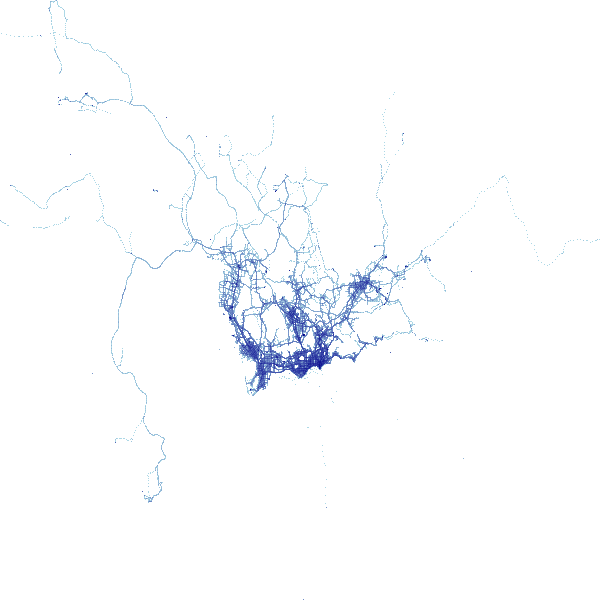### Zoom in the whole taxi trajectory dataset in Shenzhen¶

We zoom in the figure above to Shenzhen.

In :
maindt = df[abs(df.longitude -114.05) <= 0.35]
maindt = maindt[abs(maindt.latitude - 22.70) <= 0.35]

xrange = np.min(maindt['longitude']),np.max(maindt['longitude'])

yrange = np.min(maindt['latitude']),np.max(maindt['latitude'])

print (xrange,yrange)

xxrange = (113.673267, 114.646188)
yyrange  = (22.365089, 22.864404)

#cvs = ds.Canvas(x_range=xxrange, y_range=yyrange,plot_width=600, plot_height=600)
cvs = ds.Canvas(x_range=xrange, y_range=yrange,plot_width=600, plot_height=600)
agg = cvs.points(maindt,'longitude','latitude')
img

(113.7004, 114.39993) (22.35043, 23.04925)

Out: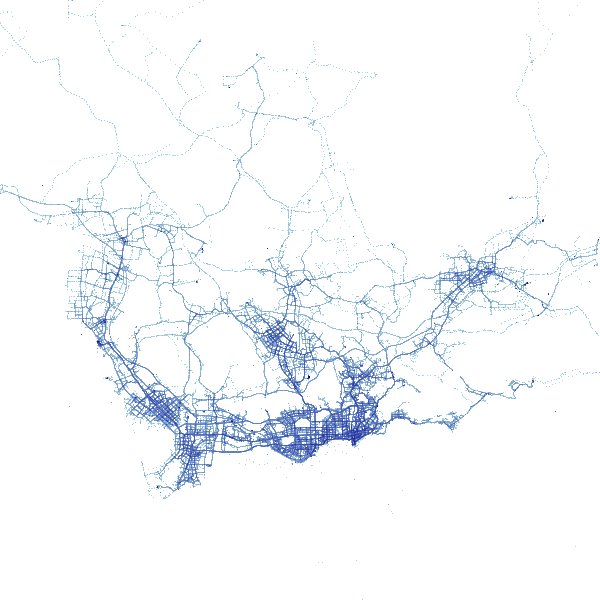### Define the histogram function¶

A histogram is a graphical display of data using bars of different heights. In a histogram, each bar groups numbers into ranges.

In :
def histogram(x,colors=None):
hist,edges = np.histogram(x, bins=100)
p = figure(y_axis_label="Pixels",
tools='', height=300, outline_line_color=None,
min_border=0, min_border_left=0, min_border_right=0,
min_border_top=0, min_border_bottom=0)
print("min: {}, max: {}".format(np.min(x),np.max(x)))
show(p)



In this case, we are defining x axis as the amount of taxi passing through for a certain area and the y axis as the amount of area.

In :
histogram(agg.values)

min: 0, max: 7018


### Define the histogram distribution using log1p¶

We have the y value to be the log1p of the aggregated value to make the histogram clearer.

In :
Greys9_r = list(reversed(Greys9))[:-2]

histogram(np.log1p(agg.values))


min: 0.0, max: 8.856376036730422

Out: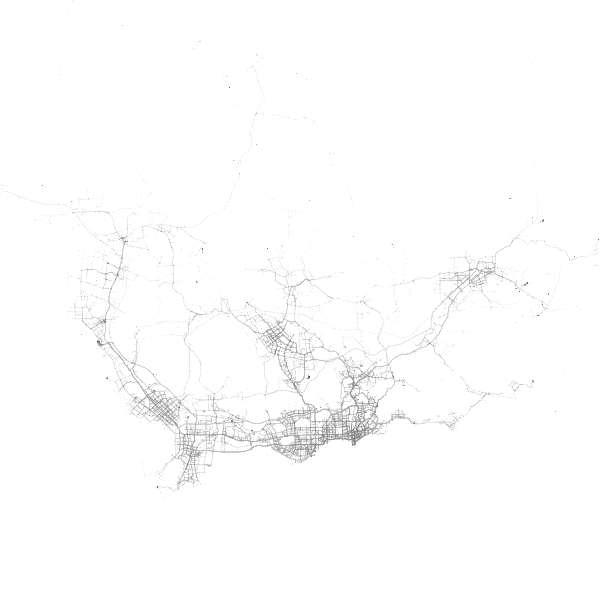### Define the histogram distribution using eq_hist¶

We have the y value to be the eq_hist of the aggregated value

In :
histogram(tf.eq_hist(agg.values))

#cmapOrange = ['darkred', 'red', 'orangered', 'darkorange', 'orange', 'gold', 'yellow', 'white']


min: 0.9276, max: 1.0

Out: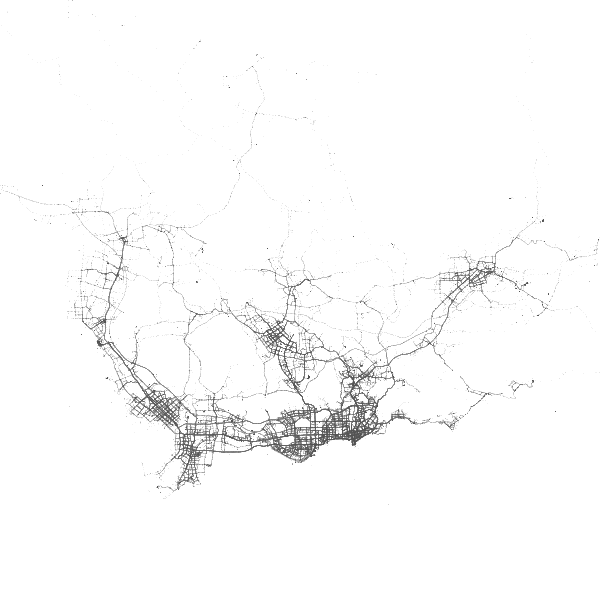### Show the heatmap using eq_hist¶

This heatmap clearly shows the area in Shenzhen where there are a lot of taxi travelling through. As we can see in the figure, the south side of Shenzhen is having more taxi trajectories than the north side of Shenzhen.

In :
#cvs = ds.Canvas(plot_width=w, plot_height=h, x_range=x_range, y_range=y_range)
cvs = ds.Canvas(plot_width=600, plot_height=600)
#agg = cvs.points(df, 'dropoff_x', 'dropoff_y',  ds.count('passenger_count'))
agg = cvs.points(maindt,'longitude','latitude')

Out: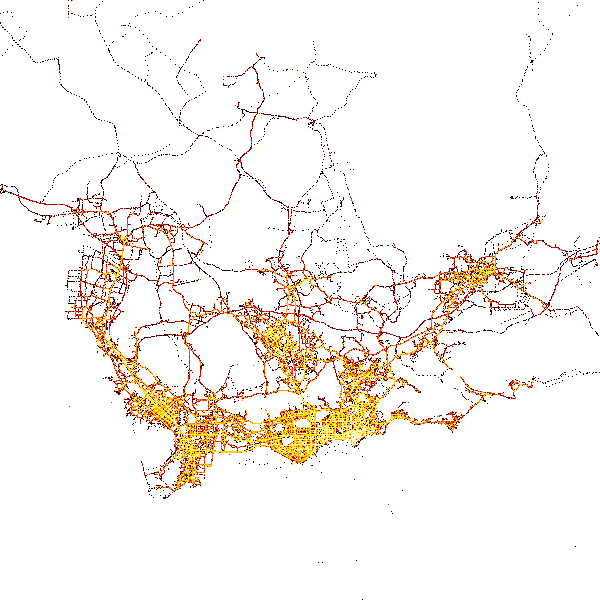### Show the heatmap with the aggregation percent greater than 90%¶

In this figure, we look at the heatmap with aggregation percent greater than 90%

In :
cvs = ds.Canvas(plot_width=600, plot_height=600)
agg = cvs.points(maindt, 'longitude','latitude')

Out: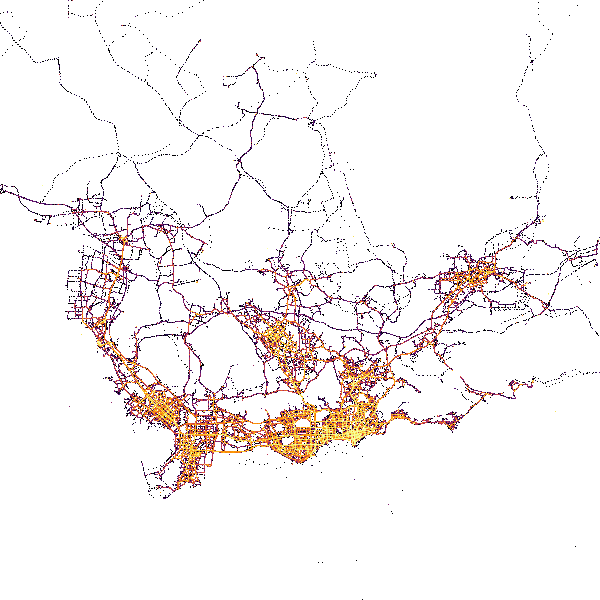## HeatMap for shenzhen taxi dataset with time information¶

Extract hour from the timestamp column to create an time_hour column, and show the example of the dataset

In :
#maindt['hour'] = maindt['timestamp'].dt.hour
maindt['hour'] = pd.to_datetime(maindt['date_time']).dt.hour.astype('category')

Out:
taxi_id date_time longitude latitude speed direction occupied other hour
0 B001B1 2009-09-23 21:32:56 114.06070 22.52170 28 0 0 0 21
1 B001B1 2009-09-23 21:33:51 114.06070 22.52170 0 0 0 0 21
2 B001B1 2009-09-23 21:34:46 114.06068 22.52165 0 0 0 0 21
3 B001B1 2009-09-23 21:35:41 114.06084 22.52182 5 0 0 0 21
4 B001B1 2009-09-23 21:36:36 114.06060 22.52258 4 0 0 0 21

### Heatmap with time information¶

First we define 24 clolrs for 24 hours. And then the heatmap with time information will be shown in different color based on the time the taxi trajectory was recorded.

In :
colors = ["#FF0000","#FF3F00","#FF7F00","#FFBF00","#FFFF00","#BFFF00","#7FFF00","#3FFF00",
"#00FF00","#00FF3F","#00FF7F","#00FFBF","#00FFFF","#00BFFF","#007FFF","#003FFF",
"#0000FF","#3F00FF","#7F00FF","#BF00FF","#FF00FF","#FF00BF","#FF007F","#FF003F",]

cvs = ds.Canvas(plot_width=600, plot_height=600)
agg = cvs.points(maindt, 'longitude','latitude',ds.count_cat('hour'))
#agg = cvs.points(df, dataset+'_x', dataset+'_y', ds.count_cat('hour'))